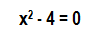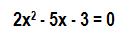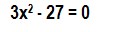# Solving Quadratic Equations with Square Roots or Graphing the Function

Instructions:

Choose an answer and hit 'next'. You will receive your score and answers at the end.

question 1 of 3

### What is the solution to the following quadratic equation?Create Your Account To Take This Quiz

As a member, you'll also get unlimited access to over 75,000 lessons in math, English, science, history, and more. Plus, get practice tests, quizzes, and personalized coaching to help you succeed.

Try it risk-free for 30 days. Cancel anytime.

### 1. Find the solution to the following quadratic equation by graphing:### 2. Find the solution to the following quadratic equation:Create your account to access this entire worksheet
A Premium account gives you access to all lesson, practice exams, quizzes & worksheets
Quizzes, practice exams & worksheets
Certificate of Completion
Create an account to get started

Review the process for solving and graphing the function of quadratic equations with square roots with this worksheet and quiz. You will need to find the solution to mathematical practice problems throughout this quiz.

## Quiz & Worksheet Goals

Get ready to demonstrate that you can:

• Solve quadratic equations
• Graph equations correctly
• Identify equations with one or more solutions

## Skills Practiced

This quiz is designed to offer you practice in these skills:

• Reading comprehension - ensure that you draw the most important information from the related lesson on solving quadratic equations
• Problem solving - use acquired knowledge to solve quadratic equations practice problems
• Knowledge application - use your knowledge to answer questions about how graphs and quadratic equations are connected

You can broaden your understanding of this subject by studying the lesson entitled Solving Quadratic Equations with Square Roots or Graphing the Function. You'll be learning more about:

• How to identify equations
• Steps involved with finding the square root of a quadratic equation
• Examples of graphed equations
Final ExamMcDougal Littell Algebra 1: Online Textbook Help
Status: Not Started
Chapter ExamMcDougal Littell Algebra 1 Chapter 10: Quadratic Equations & Functions
Status: Not Started

Support